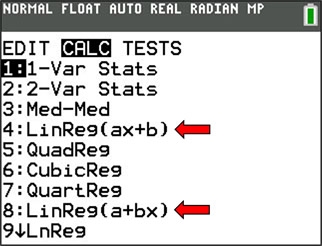LinReg(ax + b) versus LinReg(a + bx)

 Why are there TWO choices for linear regression equations on the graphing calculator? First: There is no mathematical difference between the answers produced by these two forms. There is only a difference in the order in which the answer is written. The two equations represent a difference in philosophy held by different disciplines in the mathematical community.A linear equation can be written as   y=mx+b,    y=ax+b   or even   y=a+bx.
These equations can all represent the same graphs, assuming a horizontal x-axis and a vertical y-axis.In Algebra, the equation of a line is represented by y = mx + b, where m is the slope and b is the y-intercept. Thus, algebraists prefer to maintain this format by using the form LinReg(ax + b), where a is the slope and b in the y-intercept. The format y = mx + b is a common algebraic format for the equation of a line, appearing in both high school and college texts. In Algebra, the standard form of a quadratic equation is y = ax2 + bx + c. Algebraists prefer this method as it more closely follows the standard form of a polynomial: P(x) = anxn + an-1xn-1 + ... + a1x + a0.In Statistics, the preferred equation of a line is represented by y = a + bx, where b is the slope and a is the y-intercept. (The preferred form is actually y = b0 + b1x.) Thus, statisticians prefer to maintain this format by using the form LinReg(a + bx), where a is the y-intercept and b is the slope. In advanced multi-variable statistics, equations "get richer" as terms are added at the right (that is, the powers increase moving to the right.) In Statistics, the standard form of a quadratic equation is y = a + bx + cx2. Statisticians prefer this method as it more closely follows the general form of the regression equation: y = b0 + b1x + b2x2 + ... + bnxn. Statistician will also say that they prefer this method because the variables represent the same context in each formula (that is, a is a constant in the linear equation and in the quadratic equation).

 It can be argued that there are various reasons for choosing one of these linear regression equation forms over the other. Unfortunately, it is often seen that one form is chosen to the "exclusion" of the other, with statements such as "DO NOT USE (the other form)". It is more important to understand that there are alternative ways to represent the same concepts depending upon the context in which they are used. While one form may be "preferred", both forms are beneficial. In today's interdisciplinary world, a more flexible attitude is needed. Students need to understand that parts of linear equations are commutative (such as ax + b = b + ax) and that they need to find the x-term to examine the slope (when the graph has a horizontal x-axis and a vertical y-axis). Students also need to understand that using different letters for constants and coefficients will not change the meaning of the parts of the equation (such as y = ax + b and y = a + bx, where a is the slope in the first, and b is the slope in the second). Bottom line: In high school Algebra 1, Geometry, Algebra 2, PreCalculus, Advanced Placement Calculus, and in college Algebra courses (and other college courses), LinReg(ax+b) remains the most widely used calculator form for the linear regression equation. In Advanced Placement Statistics and in College Statistics courses, LinReg(a+bx) is the more widely preferred form. If you understand "one" of these forms, you will understand the other as well. Both work!Finding Your Way Around TABLE of  CONTENTS